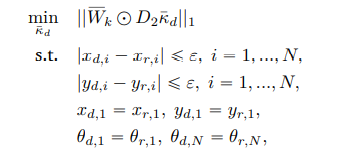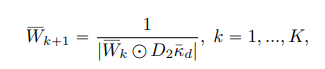# How to express iterative expression?

Hello,

I want to solve the following equation with respect to k_d in the l1 normwhere W_k starts from a vector of ones and updates itself in every iteration untill the maximum iteration K.

W_k+1 = 1/( |W_k .* (D2*Kd)| ) k=1,…,K

How is it possible to express this equation on CVX?

Thank you
All the best

I don’t understand the notation or your description of W_k, Hence, I have no idea what the stated problem is. Due to appearance in constraints, it appears that x, y, theta are optimization variables , but the image lists only Kd as an optimization variable; so I have no idea what the relations between all these things are.

As I wrote, your description of W_k is unclear, but it looks like there are products of variables (or variable expressions) in its “definition”, which would be non-convex and in violation of CVX’s rules. And the argument of the norm in the objective function likewise appears to have non-convex products. Of course, you can correct whatever misinterpretation I may be making of this very unclearly stated problem.

Your first task is to determine whether your problem is convex. In the unlikely event it is, you need to state the problem much more clearly and explicitly.

Hello again,

Since it is not allowed to add pictures more than one for a new user, i could not add the second one therefore i needed to write it by hand. So, the update rule for Wk matrix is as followsActually the problem is about mobile robots. There are some reference waypoints which are [xr,i yr,i thetar,i]. We want to minimize the second order derivate of steering angle(D2*Kd) because of the comfort issues and we also we want to take the reference trajectory into account so that my robot won’t be so far from the reference. So they are not optimization parameters, but constraints as a result of my calculated steering angle set (Kd).

Hence, i solve the optimization problem with the stated constraints and i have to update the Wk matrix. However, i could not find a way to update my Wk matrix for all iterations.

Thank you

I still don’t understand what the mathematical specification of your model is. The image for the Wk update rule doesn’t clarify anything for me.

What does it mean for the `Wk` matrix to be updated?

You need to write out (for yourself) a complete specification of the optimization problem (or is there a separate optimization problem for each iteration (whatever that is)), making clear what is input data to the problem, and what are optimization variables (or expressions involving optimization variables), and making explicit and clear the relations between all these. Then you need to determine if the optimization problem(s) is(are) convex.

If you are solving `K` different optimization problems, and for each of them, W_k (or W_{k-1} is input data, updated from the W and the optimal Kd from the previous optimization problem, then perhaps these are convex optimization problems; but that still depends on how x, y, theta are related to everything else. As it is now, there are too many unstated relationships, and I suspect they are not all convex. If there are K different optimization problems, it appears that the W update would be applied after cvx_end from one problem, and before cvx_beginning for the next optimization problem. Something like `W = initial_value;for k=1:K, cvx_begin ... cvx_end, W_is_updated;end. `W` would never be updated inside a CVX optimization problem, and therefore need not follow its rules.